## The Function Field Case

When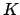is a finite separable extension of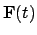, we define the divisor group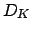ofto be the free abelian group on all the valuations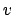. For eachthe number of elements of the residue class field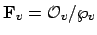ofis a power, say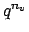, of the number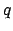of elements in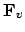. We call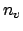the degree of, and similarly define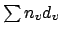to be the degree of the divisor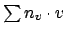. The divisors of degree 0 form a group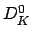. As before, the principal divisor attached to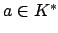is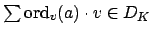. The following theorem is proved in the same way as Theorem 21.2.2.

Theorem 21.2.3   The quotient ofmodulo the principal divisors is a finite group.

William Stein 2004-05-06